# distribution

(redirected from T-distribution)
Also found in: Dictionary, Thesaurus, Medical, Legal, Financial.
Related to T-distribution: T-test

## distribution

distribution, in economics, the allocation of a society's total wealth among various economic groups. Distribution, in that sense, does not refer to the physical marketing or circulation of goods, which is part of the process of exchange, but to the relative well-being and economic wealth of persons and groups. By classifying people according to their share of the distribution—usually by means of relative income—a picture of society's stratification, and thus its structure, may emerge. Italian economist Vilfredo Pareto developed (1897) one of the best–known theories on the subject, arguing that a pattern of income distribution is evident throughout history, in all societies. Inequalities in distribution are related to inequalities in political power; in most societies, the economically dominant strata tend also to be politically dominant. The division of labor, which necessitates exchange, causes various problems of distribution. Inequalities in distribution among industrial groups making a common product are explained by the relative number employed in each group when compared to the value of what they produce or to the relative amount they get for a specific amount of work. Unequal distribution arises also from inequality in reward to those in the same industrial classification (capitalist, manager, or laborer). The distribution of wealth between the capitalist and manager on the one hand and the laborer on the other has been a major source of social strife in the Western world since the French Revolution, and has been a particularly important theme in the writings of Karl Marx and his followers. Labor unions (see union, labor), through the use of political and economic pressure, have striven for increased wages. The distribution of the world's wealth has, since World War II, become a major issue in international politics, especially as those nations that had previously been the colonized suppliers of raw materials to the industrialized countries have gained political independence and embarked on development programs. In the United States, the National Bureau of Economic Research has done important studies on the distribution of wealth among economic groups.

### Bibliography

See F. Levy, Dollars and Dreams: The Changing American Income Distribution (1988); K. M. Perkins, Production, Distribution, and Growth in Transitional Economies (1988).

The following article is from The Great Soviet Encyclopedia (1979). It might be outdated or ideologically biased.

## Distribution

a term used in structural linguistics, especially in American descriptive linguistics. The distribution of a given element is usually understood as the sum of all environments in which it is encountered—that is, the sum of all the different positions of an element with respect to the positions of the other elements. The concept of distribution reflects the fact that each linguistic unit (with the exception of the sentence) has a more or less limited capacity to combine with other similar units.

Four types of distribution are distinguished. The first type is when two elements are never found in the same position; this type, called complementary distribution, is characteristic of variants of the same unit (thus, the more open and more closed vowels in the words ded and det’ , the former of which is found in Russian speech before hard consonants and the latter before soft consonants; they are variants of the phoneme [e]). The second type is when two elements are found in identical environments—in this case it is a question either of “contrastive distribution,” which characterizes functionally different units (for example, two sounds, the replacement of one of which by the other entails a difference in meaning) that belong to a single class, or of “free alternation” of optional variants of the same unit (as the trilled and uvular untrilled r in French and the endings -oi and -oiu in the instrumental singular in Russian). The third type is when the set of environments in which there is one element includes a set of environments in which the other element is found—this type of “contrastive distribution” is characteristic of functionally opposed units, one of which is usually described as having a positive marker, or as being marked, and the other as having a negative marker, or as being unmarked (thus, the distribution of Russian unvoiced consonants is more extensive than the distribution of voiced consonants, since the latter do not occur in final position). The fourth type is when sets of environments of two units or two classes of units partially intersect (thus, for example, in Czech the distribution of r and l, which belong to the consonant class, partially intersects with the distribution of vowels—that is, they can be the central element of a syllable, such as in vlk, “;wolf,” and prst, “finger”).

### REFERENCE

Gleason. H. Vvedenie v deskriptivnuiu lingvistiku. Moscow, 1959. (Translated from English.)

T. V. BULYGINA

## Distribution

one of the phases (stages) of social reproduction, the link between production and consumption. The share (proportion) of the producers in the realization and use of the aggregate social product and the national income is revealed in the process of distribution. This process is preceded by the distribution of the means of production among the sectors of the national economy and among enterprises, as well as by the distribution of the members of society in the various types of production. Production is the most important and the determining factor in the unity of the components of social reproduction.

K. Marx noted that “in the process of production, the members of society adapt (create, transform) the products of nature to human needs; distribution establishes the proportion in which each individual participates in the produced…. Distribution determines the ratio (quantity) in which the products are made available to individuals” (in K. Marx and F. Engels, Soch.,, 2nd ed., vol. 12, pp. 714, 715).

Each mode of production determines its own forms of distribution. Marx wrote: “The structure of distribution is completely determined by the structure of production. Distribution itself is a product of production not only in terms of content, for the results of production alone can be distributed, but also in terms of form, for a certain method of participation in production determines the special form of distribution, the form in which there is participation in distribution” (ibid., p. 721).

The character of production determines the character of distribution, but distribution also has an active influence on production, the growth of which it may either promote or impede, for example. Moreover, distribution may ensure the development of certain sectors and restrain the development of others, and it may alter the relationship between industrial and personal consumption by increasing the share of the product for industrial consumption and reducing the share for personal consumption, and vice versa.

Under capitalism, distribution has an antagonistic character. A significant share of the gross national product and the national income of the capitalist countries is appropriated by the financial oligarchy, the representatives of which control gigantic monopolistic corporations (seeMONOPOLIES, CAPITALIST). For example, more than 50 percent of the US national income goes to the capitalists. (This figure takes into account the redistribution of the national income.)

A portion of the gross national product of a capitalist society is allocated by the ruling class to pay for wage labor. The distribution of consumer goods among the exploited, in accordance with the social character of capitalism, determines the share of each worker in the aggregate wages fund, depending on the value of labor power. Taking advantage of unemployment, the capitalists try their utmost to further reduce the workers’ wages below the value of labor power.

The inverse effect of distribution on production under capitalism consists primarily in the fact that private capital, depending on its magnitude, varies in its capacity for further expansion by means of profit. Large-scale capital becomes even larger and stronger and preys on medium- and small-scale capital, which often cannot withstand the competitive struggle (seeCOMPETITION). The distribution of consumer goods among the workers does not make it possible for them to free themselves from the oppression of capital. Deprived of the means of production, the workers are forced to sell their labor power to the capitalists. Under state-monopoly capitalism, the monopolies intensify the exploitation of their own workers and of other strata of the working people, including the peoples of dependent nations (seeNEOCOLONIALISM).

Under socialism, with the dominance of social ownership, the means of production are distributed among the sectors of the national economy and among enterprises in accordance with the needs of expanded socialist reproduction, with the aim of providing for continuous improvement in the material and cultural level for the comprehensive development of the entire society and all of its members. The means of production are distributed according to the plans for material and technical supply.

Socialism and capitalism also differ fundamentally in the distribution of labor resources. Under socialism, the training of specialists and their distribution in the various spheres of production have a planned character. Nonetheless, consideration is given to the desire of members of society to work in a chosen occupation at a particular enterprise. Economic incentives such as wage differentiation are extensively used in the distribution of labor resources among the sectors of the natural economy and among various regions.

Social ownership of the means of production results in the distribution of the social product and the national income in the interests of the working people. The socioeconomic meaning of socialist production, which is fundamentally new in comparison to that of capitalist production, gives rise to fundamentally new proportions and forms of distribution. In the first phase of the communist mode of production, consumer goods and services are distributed according to the quantity and quality of labor performed by each worker. This is necessary because under socialism, labor has not yet become man’s primary vital need, nor has it been transformed into a custom of working without consideration for renumeration. Under socialism, labor requires a material incentive. Moreover, the level of productivity of social labor and the volume of production cannot yet provide an abundance of material goods and services. Therefore, society must exercise control over the measure of labor and consumption of each of its members. Labor is controlled and encouraged by means of the law of distribution according to labor.

As Marx emphasized, under socialism distribution has specific characteristics: “In a society based on the principles of collectivism and on the common ownership of the means of production… each individual producer will receive from society, after all deductions have been made, just as much as he produced for it” (ibid., vol. 19, p. 18). According to V. I. Lenin, one of the most important principles of distribution under socialism is “an equal amount of products for an equal amount of labor” (Poln. sobr. soch., 5th ed., vol. 33, p. 94). In addition to the wages received by the members of a socialist society according to their labor, a portion of the assets is paid to them as bonuses drawn from the material incentive fund for the employees of an enterprise. This fund is made up of the profits earned by an enterprise. Consequently, the size of the fund and of the bonuses depends on the results of the work of the enterprise collective as a whole.

Distribution according to labor stimulates the fulfillment and overfulfillment of production plans and fosters the workers’ desire to work better, to raise labor productivity, and to improve product quality. It also encourages the workers to improve their skills, because more highly skilled labor is paid higher rates. Under socialism a portion of the means of subsistence is distributed through the social consumption fund. Under the conditions of socialism, this form of distribution supplements distribution according to labor, and to some degree, it has no relation to the individual’s share of labor in social production. As socialist production develops, distribution of the means of subsistence through the social consumption fund assumes increasing significance and contributes to greater social equality.

At the higher phase of the communist formation—under full communism—consumer goods and services will be distributed according to the principle “from each according to his ability, to each according to his needs” (K. Marx, in Marx and Engels, Soch., 2nd ed., vol. 19, p. 20). Referring to the higher phase of communism, Lenin wrote: “There will be no need for society, in distributing products, to regulate the quantity to be received by each; each will take freely ‘according to his needs’” (Poln. sobr. soch., 5th ed., vol. 33, pp. 96-97). Distribution according to needs will become possible at a higher stage of development of the productive forces, which will provide an abundance of material goods and services.

### REFERENCES

Iz rukopisnogo nasledstva K. Marksa. K. Marx and F. Engels, Soch., 2nd ed., vol. 12, pp. 714–24.
Marx, K. Kritika Gotskoi programmy. Ibid., vol. 19, pp. 18-21.
Lenin, V. I. Gosudarstvo i revoliutsiia. Poln. sobr. soch., 5th ed., vol. 33, pp. 94-97.

G. N. KHUDOKORMOV

## Distribution

a fundamental concept of probability theory and mathematical statistics. The probability distribution of a random variable—that is, of a quantity whose assumption of a particular numerical value depends on chance—can be defined by indicating the possible values of the variable and the corresponding probabilities of these values. Consider, for example, the number m of spots on the upward face of a die that has been tossed. The distribution of probabilities pm is given in Table 1. Similarly, the distribution of any random variable X whose possible values form a finite or infinite sequence can be defined by indicating the values

x1, x2, …, xn, …

and the corresponding probabilities

p1, p2, …, pn, …

Here, the probabilities pm must be positive, and their sum must be equal to unity. Such a distribution is said to be discrete. The Poisson distribution is an example of a discrete distribution; it is defined by the probabilities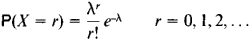where λ > 0 is a parameter.

Table 1
Possible values of m.............123456
Corresponding probabilities pm ......1/61/61/61/61/61/6

It is not always possible, however, to define a distribution by indicating the possible values xn and the corresponding probabilities pn. For example, if a variable is distributed uniformly over the closed interval [–½, + ½], as are rounding errors in the measurement of continous variables, each individual value has zero probability. The distribution of such random variables can be defined by indicating the probability that the random variable X takes on a value from any given interval. When there exists a function px (x) such that the probability of X falling within any interval (a, b) is given by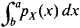the distribution of the random variable is said to be continuous. The function px (x) is called the probability density function. The probability density function is nonnegative and possesses the property that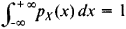In the above case of a uniform distribution over the closed interval,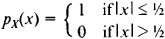The most important type of continuous distribution is the normal distribution with density function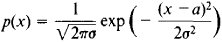where a and σ > 0 are parameters.

The distributions of random variables are not limited to the discrete and continuous types and may have a more complex nature. For this reason, a description of distributions that covers all cases is desirable. Such a description can be obtained, for example, by means of what is called the distribution function Fx (x). For any given x, this function has a value equal to the probability P{X < x} of the random variable X taking on a value less than x, that is,

Fx (x) = P{X < x)

The distribution function is a nondecreasing function of x that goes from 0 to 1 as x increases from - ∞ to + ∞. The probability that X takes on a value from some half-open interval [a, b) is equal to the probability that X satisfies the inequality aX < b. In other words, the probability is equal to

F(b) – F(a)

Examples. (1) Suppose E is some event with probability of occurrence p, where 0 < p < 1. Then the number μ of occurrences of the event E in n independent observations is a random variable that takes on the values m = 0, 1, 2, …, n with probabilities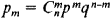where q = 1 – p. This distribution is called the binomial distribution. It follows from the Laplace theorem that for large n the binomial distribution (see Figure 1) can be approximated by the normal distribution.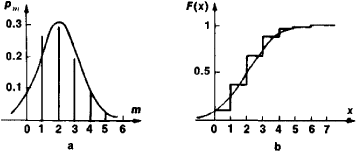Figure 1.

Note on Figure 1. Binomial distribution: (a) probabilities, (b) distribution function (n = 10, p = 0.2); the smooth curves represent a normal approximation of the binomial distribution

(2) The number of observations up to and including the first occurrence of the event E in example (1) is a random variable that takes on all the integral values m = 1, 2, 3,… with probabilities

pm = qm-1p

This distribution is called the geometric distribution, since the sequence {pm} is a geometric progression (see Figure 2).

(3) A distribution whose density function p(x) is equal to ½h over some interval (ah, a + h) and to zero outside this interval is called a uniform distribution. The corresponding distribution function increases linearly from 0 to 1 as x increases from a - h to a + h (see Figure 3).

Other examples of probability distributions are the Cauchy distribution, Pearson curves, the multinomial distribution, the exponential distribution, the χ2 distribution, and Student’s distribution.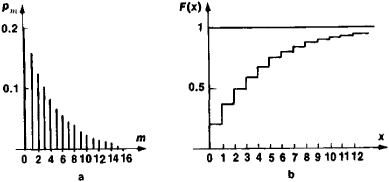Figure 2. Geometric distribution: (a) probabilities pm = qm-1p, (b) distribution function (p = 0.2)

If the random variables X and Y are connected by the relation Y = f(X), where f(x) is a given function, then the distribution of Y can be expressed rather simply in terms of the distribution of X. For example, if X has a normal distribution and Y = ex, then Y has what is called a lognormal distribution (see Figure 4) with density function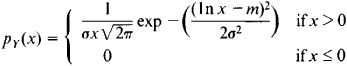The formulas relating the distributions of the random variables X and Y become particularly simple when Y = aX + b, where a and b are constants. Thus, if a> 0,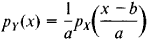and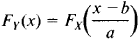Instead of a complete description of a distribution by means of, for example, the probability density function or the distribution function, often a small number of characteristics, characteristic quantities, are given that indicate the most typical, in some sense, values of the random variable or the degree of variation of the values of the random variable about some typical value. The most useful of these characteristics are the mathematical expectation (mean value) and the variance. The mathematical expectation EX of a random variable X with a discrete distribution is defined as the sum of the series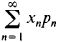provided that this series converges absolutely. For a random variable X having a continuous distribution with density px (x) the mathematical expectation is determined by the formula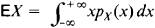provided that this integral converges absolutely. If Y = f(X), EY may be calculated in either of two ways. For example, if X and Y have a continuous distribution, then, on the one hand, by definition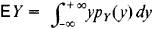and, on the other hand, it can be proved that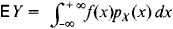The variance DX is defined as follows:

DX = E (X - EX)2

For example, in the case of a continuous distribution,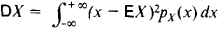The probability distribution has much in common with the distribution of a mass on a line. Thus, if we have a random variable X that assumes the values x1, x2, …, xn with probabilities p1, p2, …, pn, we can associate with X a distribution of masses such that masses equal to pk are located at the points xk. The formulas for EX and DX here are the same as the formulas for the center of gravity and the moment of inertia, respectively, of this system of mass points. The quantitative characteristics of distributions are discussed in greater detail in the articles QUANTILE; MEDIAN: MODE; EXPECTATION. MATHEMATICAL; DEVIATION; and ROOT-MEAN-SQUARE DEVIATION.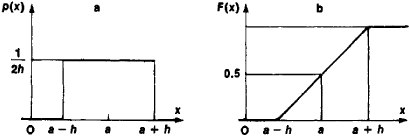Figure 3. Uniform distribution: (a) probability density function, (b) distribution function

If several independent random variables are added together, their sum will be a random variable whose distribution depends only on the distributions of the summands. In general, this is not the case for a sum of dependent random variables. Suppose, for example, two independent random variables, each with a continuous distribution, are summed. For such a sum the following formula holds: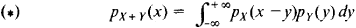Under extremely broad assumptions, the distribution of a sum of independent random variables tends to a limiting distribution—for example, the normal distribution—as the number of random variables tends to infinity. Explicit formulas such as (*), however, are in practice unsuitable for establishing this fact. The proof is consequently done indirectly, generally through the use of characteristic functions.

Statistical distributions and their connection to probabilities. Suppose n independent observations are made of the random variable X having the distribution function F(x). The statistical distribution of the results of the observations can be defined by indicating the observed values x1, x2, …, xr of the random variable X and the corresponding frequencies h1, h2, …, hr, where the frequencies are the ratios of the number of observations in which the given value appears to the total number of observations. For example, suppose that in 15 observations the value 0 is observed eight times, the value 1 five times, the value 2 once, and the value 3 once. The corresponding statistical distribution is given in Table 2. The frequencies are always positive, and their sum is always unity.

Table 2
Observed values xm ..........0123
Corresponding frequencies hm .....8/151/31/151/15

If the term “probability” is replaced by the term “frequency,” many of the definitions given above for probability distributions can be applied to statistical distributions. Thus, suppose that x1, x2, … xr, are the observed values of X and that h1, h2, …, hr are the frequencies of these observed values. The mean and variance corresponding to the statistical distribution—that is, the sample mean and sample variance—are then defined by the equations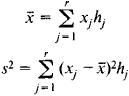and the corresponding distribution function, which is called the empirical distribution function, is given by the equation

F*(x) = nx/n

where nx is the number of observations whose result is less than x. The statistical distribution and its characteristics can be used to approximate the theoretical distribution and its characteristics. If, for example, X has finite mathematical expectation and finite variance, then for arbitrary ε > 0 the inequalities

ǀx̄ – EXǀ < ε

ǀs2DXǀ < ε

are satisfied for sufficiently large n with probability arbitrarily close to unity. Thus, x̄ and s2 are consistent estimates of EX and DX, respectively. The Soviet mathematician V. I. Glivenko proved that for any ε > 0 the probability of the inequality

ǀF*n (x) – F(x)ǀ < ε

approaches unity for all x as n tends to infinity. A more precise result was established by the Soviet mathematician A. N. Kolmogorov (seeNONPARAMETRIC METHODS).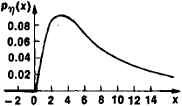Figure 4. Density of lognormal distribution (m = 2, σ = 1)

Multivariate distributions. Suppose X and Y are random variables. With every pair (X, Y) we may associate a point Z in the plane with coordinates X and Y whose position depends on chance. The joint distribution of X and Y can be defined by indicating the possible positions of Z and the corresponding probabilities. As above, two basic types of distributions may be distinguished—discrete and continuous.

In discrete distributions the possible positions of Z form a finite or infinite sequence. The distribution can be defined by indicating the possible positions of Z

z1, z2, …, zn, …

and the corresponding probabilities

p1, p2, …, pn, …

Continuous distributions can be specified by the probability density p (x, y), which has the property that the probability of Z falling in some region G is given by the double integral

Gp (x, y) dx dy

An example is the bivariate normal distribution with density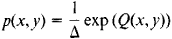Here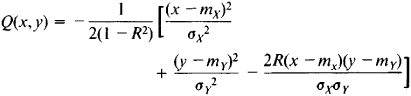where

mx = EX my = EY

are the mathematical expectations of X and Y and

σx2 = E (XmX)2 σy2 = E (XMY)2

are the variances of X and Y. The quantity Δ is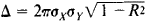Finally, R is the correlation coefficient between X and Y: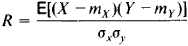Probability distributions in spaces of three or more dimensions can be treated in a similar manner. Besides the concept of correlation, another concept important in the study of multivariate distributions is that of regression.

The possibility of further generalizations and the connection between the concept of the measure of a set and the concept of distribution are discussed in the article PROBABILITY THEORY.

### REFERENCES

Gnedenko, B. V. Kurs teorii veroiatnostei, 5th ed. Moscow, 1969.
Cramer, H. Matematicheskie melody statistiki. Moscow, 1948. (Translated from English.)
Feller, W. Vvedenie v teoriiu veroiatnostei i ee prilozheniia, 2nd ed., vols. 1-2. Moscow, 1967. (Translated from English.)
Bol’shev, L. N., and N. V. Smirnov. Tablilsy matematicheskoi statistiki, 2nd ed. Moscow, 1968.

Iu. V. PROKHOROV

## distribution

[‚dis·trə′byü·shən]
(industrial engineering)
All activities that involve efficient movement of finished products from the end of the production line to the consumer.
(mathematics)
An abstract object which generalizes the idea of function; used in applied mathematics, quantum theory, and probability theory; the delta function is an example. Also known as generalized function.
(statistics)
For a discrete random variable, a function (or table) which assigns to each possible value of the random variable the probability that this value will occur; for a continuous random variable x, the monotone nondecreasing function which assigns to each real t the probability that x is less than or equal to t. Also known as distribution function; probability distribution; statistical distribution.
McGraw-Hill Dictionary of Scientific & Technical Terms, 6E, Copyright © 2003 by The McGraw-Hill Companies, Inc.

## distribution

The movement of freshly mixed concrete toward the point of placement, either by motorized tools or by hand.
McGraw-Hill Dictionary of Architecture and Construction. Copyright © 2003 by McGraw-Hill Companies, Inc.

## distribution

1. Commerce the process of physically satisfying the demand for goods and services
2. Economics the division of the total income of a community among its members, esp between labour incomes (wages and salaries) and property incomes (rents, interest, and dividends)
3. Law the apportioning of the estate of a deceased intestate among the persons entitled to share in it
4. Law the lawful division of the assets of a bankrupt among his creditors
5. Engineering the way in which the fuel-air mixture is supplied to each cylinder of a multicylinder internal-combustion engine
Collins Discovery Encyclopedia, 1st edition © HarperCollins Publishers 2005

## distribution

(software)
A software source tree packaged for distribution; but see kit.

## distribution

(messaging)
A vague term encompassing mailing lists and Usenet newsgroups (but not BBS fora); any topic-oriented message channel with multiple recipients.

## distribution

(messaging)
An information-space domain (usually loosely correlated with geography) to which propagation of a Usenet message is restricted; a much-underused feature.

## distribution

(1) Software ready to install. It often refers to a "Linux distribution," which is the Linux OS combined with installation programs, documentation and various utilities in one package. See Linux distribution.

(2) An English word that means "dissemination" or delivering something.
Copyright © 1981-2019 by The Computer Language Company Inc. All Rights reserved. THIS DEFINITION IS FOR PERSONAL USE ONLY. All other reproduction is strictly prohibited without permission from the publisher.
References in periodicals archive ?
From the t-distribution with df = 18, we obtain the p-value of 0.0001, which shows strong evidence to reject the null hypothesis.
In the RBN with T-distribution function outermost layer nodes, the corresponding conditional probability of outermost layer nodes and hidden layer nodes can be represented as:
Let us study performances of the Edgeworth expansion  and the Gaussian mapping  comparing them with the result of the bivariate t-distribution .
It was found that it was possible to fit MGHD models to joint returns, with this model narrowly outperforming the multivariate Student's t-distribution with the next best fit.
where [[PHI].sub.v-2] (.) is the cumulative distribution function of a standard t-distribution with u-2 degrees of freedom, and [[eta].sup.*] is the solution to the following equation
Expanded uncertainty for standard loss measurement: Since the effective degree of freedom is more than 30, from t-distribution table at 95% CL, the coverage factor K = 1.96
For a finite number of degrees of freedom, k is estimated as the t-factor from the Student's t-distribution based on the number of degrees of freedom and about a 95% confidence interval.
To do this, we estimate the degrees of freedom of the t-distribution, v, as part of the analysis.
With the previously stated distributional assumptions, t has a central t-distribution if [Rho] = [[Rho].sub.goal], with cumulative distribution function (cdf) T and inverse cdf [T.sup.-1].
Thus, [H.sub.0] would be rejected if and only if t < t([gamma]; [[delta].sub.0], f), where t([gamma]; [[delta].sub.0], f) is the [gamma]th lower percentage point of the noncentral t-distribution with noncentrality parameter [[delta].sub.0] (Eq.
The error for an estimated average is determined assuming that (X - [micro])/([sigma]/[square root]n) follows the t-distribution (where [micro] = the population average, X = the sample average, [sigma] = the sample standard deviation, n = the sample size).

Site: Follow: Share:
Open / Close